# Fourier Series

This section is devoted to periodic extensions of functions defined on some finite interval. Since Fourier series provide periodic extensions, we need to compare it with corresponding periodic extension of the original function.

Introduction to Linear Algebra with Mathematica

# Periodic Extension

Let a and b be real numbers such that a < b, and let f be a real-valued function $$f\, : \, (a,b] \mapsto \,\mathbb{R} .$$ The function $$F\, : \, \mathbb{R} \mapsto \,\mathbb{R}$$ defined by
$F(x) = f \left( x - \left\lfloor \frac{x-a}{b-a} \right\rfloor \left( b-a \right) \right) , \quad x \in \mathbb{R} ,$
is called the periodic extension of f.
Here $$\lfloor {\text a} \rfloor$$ is the floor of a real number a, which is the largest integer that does not exceed a. There are several known types of periodic extensions, two of which will be discussed later. Periodic extension is very important topic in electrical engineering, called rectification. A rectifier is an electrical device that converts alternating current (AC), which periodically reverses direction, to direct current (DC), which flows in only one direction. Currently, there are two known rectifiers: the half-wave rectifier and full-wave rectifier. While three-phase rectifiers are more common in industry, we are not going to consider them now. Instead, we give mathematical definitions of electrical counterparts that are related to periodic extensions.
Let $$f\, : \, (a,b] \mapsto \,\mathbb{R}$$ be a real-valued function defined on a finite interval. Its full-wave rectifier is a periodic function with period b-a, which is obtained by periodic extension of the function f from the original interval. Its half-wave rectifier is a periodic extension with period 2(b-a) of the function
$F(x) = \begin{cases} f(x) , & \ \mbox{ if } a < x \le b , \\ 0 , & \ \mbox{ if } b < x \le 2b-a . \end{cases}$
Let a and b be real numbers such that a < b, and let f be a piecewise continuous real-valued function $$f\, : \, (a,b] \mapsto \,\mathbb{R} .$$ The function $$F\, : \, \mathbb{R} \mapsto \,\mathbb{R}$$ is said to be the Fourier periodic extension of f if it is its periodic extension with period b-a satisfies the following conditions:
$F_{Fourier} (x) = \begin{cases} F \left( x \right) , & \quad \mbox{if F(x) is continuous at } x , \\ \frac{1}{2} \left[ F(x+0) + F(x-0) \right] , & \quad \mbox{if F is discontinuous at } x, \end{cases} \qquad x \in \mathbb{R} .$

Here the expression F(a + 0) means the limit value from the right: $$F(a+0) = \lim_{\epsilon \mapsto 0>0} F(a+\epsilon ) .$$ Similarly, $$F(a-0) = \lim_{\epsilon \mapsto 0>0} F(a-\epsilon ) .$$ The condition at the point of discontinuity follows from the fact that a Fourier series, if it converges at this point, is equal to the average values of left and right limits. Therefore, it does not matter what value f(a) is assigned initially at the point of discontinuity x = a, its Fourier series will define it to be $$\frac{1}{2} \left[ f(a+0) + f(a-0) \right] .$$

Example: Consider the function $$f(x) = 1-x$$ on the interval [0,1]. To define its periodic extension, we use Mathematica command Mod:

periodicExtension[func_ , period_, offset_] := func[Abs[Mod[t, period, offset]]];
f[t_]=1-t;
f10=periodicExtension[f,1,0];
Plot[f10,{t,0,4}, PlotStyle -> {Thick, Red}, PlotLabels ->"Expressions"] f1 = periodicExtension[f,1];
Plot[f1,{t,0,4}, PlotStyle -> Thick, ColorFunction -> Function[{x,y}, Hue[y]]]
f12 = periodicExtension[f,1, 1/2];
Plot[f12,{t,0,4}, PlotStyle -> Directive[Opacity[0.5], Thick], Mesh ->20, MeshFunctions -> {#1 &}, MeshShading -> {Red, Green}]
f1m1 = periodicExtension[f,1, -1];
Plot[f1m1,{t,0,4}, PlotStyle ->Orange, Mesh -> All]
f21 = periodicExtension[f,2,1];
Plot[f21,{t,0,4}, PlotStyle->Thick, ColorFunction -> Function[{x,y}, Hue[x]], ColorFunctionScaling->True]
f20 = periodicExtension[f,2,0];
Plot[f20,{t,0,4}, PlotStyle->Thick, Epilog -> {PointSize[0.03], Point[{1,0}], Point[{3,0}]}]
f2m1 = periodicExtension[f,2,-1];
Plot[f2m1,{t,0,4}, PlotStyle -> Thick, Mesh ->{Range[0,1,1/4]}, MeshStyle ->PointSize[Medium]]

To plot half-wave rectifiers, we need to define another piecewise continuous function

$g(x) = \begin{cases} 1-x , & \ \mbox{ if } 0 < x \le 1, \\ 0 , & \ \mbox{ if } 1 \le x < 2. \end{cases}$
g[x_] = Piecewise[{{1-x, 0<x<1}, {0,1<x<2}}]
Then we plot half-wave rectifier of our function.
g10=periodicExtension[g,2,0];
Plot[g10,{t,0,4}, PlotStyle -> {Thick, Red}, PlotLabels ->"Expressions"]
Similarly, we get other extensions.
g4 = periodicExtension[g,4];
Plot[g4,{t,0,4}, PlotStyle -> Thick, ColorFunction -> Function[{x,y}, Hue[y]]]
g2m2 = periodicExtension[g,2, -2];
Plot[g2m2, {t,0,4}, PlotStyle -> Directive[Opacity[0.5], Thick], Mesh ->20, MeshFunctions -> {#1 &}, MeshShading -> {Red, Green}]
g30 = periodicExtension[g,3,0];
Plot[g30 ,{t,0,4}, PlotStyle -> Thick, Mesh ->{Range[0,1,1/4]}, MeshStyle ->PointSize[Medium]]

In each example below we start with a function defined on an interval, plotted in blue; then we present the periodic extension of this function, plotted in other color; then we present the Fourier expansion of this function, which is a Fourier periodic extension of the given function. The last figure in each example shows in one plot the Fourier expansion and the approximation with the partial sum with 20 terms of the corresponding Fourier series.

Example: Consider the following functions:

• $$f_1 (x) = \mbox{sign}(x)\,: \, (-1,1] \mapsto \, \mathbb{R})$$
• a = Plot[{Sign[t]}, {t, -2, 2}, PlotStyle -> {Thick, Blue}, AspectRatio -> 1/2]
b = Graphics[{Blue, Thick, Circle[{0, 0}, 0.02]}, AspectRatio -> 1]
Show[a, b]
However, it is not what we want because the signum function is defined on the infinite real line $$(-\infty , \infty ) ,$$ but we need its periodic extension with period 2.
a = Graphics[{Red, Arrowheads[0.05], Arrow[{{-1, -1}, {0, -1}}]}]
b = Graphics[{Red, Arrowheads[0.05], Arrow[{{1, 1}, {0, 1}}]}]
c = Graphics[{Red, Thick, Circle[{0, 0}, 0.05]}, AspectRatio -> 1/2]
b2 = Graphics[{Red, Arrowheads[0.05], Arrow[{{3, 1}, {2, 1}}]}]
a1 = Graphics[{Red, Arrowheads[0.05], Arrow[{{1, -1}, {2, -1}}]}]
c2 = Graphics[{Red, Thick, Circle[{2, 0}, 0.05]}, AspectRatio -> 1/2]
a3 = Graphics[{Red, Arrowheads[0.05], Arrow[{{3, -1}, {4, -1}}]}]
ac = Graphics[{Red, Thick, Circle[{-1, -1}, 0.05]}, AspectRatio -> 1/2]
ac1 = Graphics[{Red, Thick, Circle[{1, -1}, 0.05]}, AspectRatio -> 1/2]
ac3 = Graphics[{Red, Thick, Circle[{3, -1}, 0.05]}, AspectRatio -> 1/2]
c4 = Graphics[{Red, Thick, Circle[{4, 0}, 0.05]}, AspectRatio -> 1/2]
b4 = Graphics[{Red, Arrowheads[0.05], Arrow[{{5, 1}, {4, 1}}]}]
Show[a, b, c, c2, b2, a1, a3, ac, ac1, ac3, c4, b4]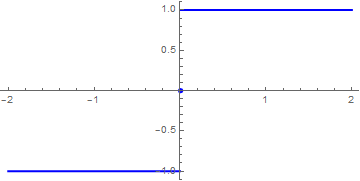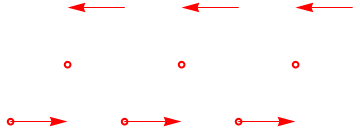Therefore, we define a periodic extension of the signum function:
fbas[x_] := -1 /; -L <= x < 0 (*square wave*)
fbas[x_] := 1 /; 0 <= x < L
L = 1;
f[x_] := fbas[Mod[x, 2*L, -L]]
SetOptions[Plot, ImageSize -> 300];
Plot[f[x], {x, -3*L, 3*L}, PlotRange -> {-1.2, 1.2}, AxesLabel -> {"x", "f(x)"}, PlotStyle -> Thickness[0.008]]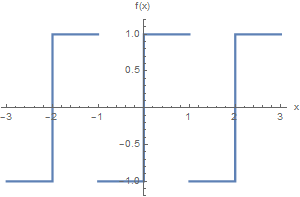The function Mod[x, 2*L, -L]
(2) calculates the remainder on dividing (1) by 2*L,
(3) subtracts L from (2). This has the effect of shifting the original x by ±2L until it falls into the range [-L,L). This modified x is then used as the argument of the original function f[x].

We plot the periodically extended function over three periods -- that is over [-3L, 3L].
Strictly speaking the vertical lines shown on the graph at the discontinuity are not part of the graph. In some ways they make it easier to visualize the discontinuous function, but on those days when we are feeling fussy, we can exclude those segments. This is done by an Option for plot called Exclusions. The plot without the vertical lines is constructed below. The argument of the Option Exclusions is a list of points to be excluded.

Plot[f[x], {x, -3*L, 3*L}, PlotRange -> {-1.2, 1.2}, AxesLabel -> {"x", "f(x)"}, Exclusions -> {-2.0, -1.0, 0.0, 1.0, 2.0}, Epilog -> {Red, PointSize[Medium], Point[{{-2, 0}, {-1.0, 0}, {0, 0}, {1, 0}, {2, 0}, {3, 0}}]}]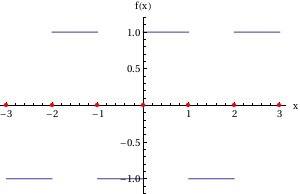Then we plot Fourier approximation with 20 terms:
f = FourierTrigSeries[Sign[x], x, 20, FourierParameters -> {1, Pi}]
A=Plot[f, {x, -5, 5}, PlotStyle -> {Thick, Orange}, AspectRatio -> 2/5]
cm1 = Graphics[{Red, Thick, Disk[{-1, 0}, 0.07]}, AspectRatio -> 1/4]
Show[A, c4, c3, c2, c1, c0, cm1, cm2, cm3, cm4]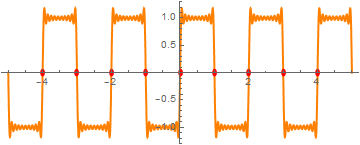because Fourier coefficients are
$a_k = \int_{-1}^1 \mbox{sign} (x)\,\cos \left( k\pi x \right) {\text d}x = 0, \qquad b_k = \int_{-1}^1 \mbox{sign} (x)\,\sin \left( k\pi x \right) {\text d}x = \frac{2}{k\pi} \left[ 1- (-1)^k \right] = \frac{4}{k\pi} \times \begin{cases} 1, & \ \mbox{if k is odd}, \\ 0, & \ \mbox{if k is even} . \end{cases}$
Then
$\mbox{sign}(x) = \frac{4}{\pi}\, \sum_{n\ge 1} \frac{1}{2n-1} \,\sin (2n-1)\pi x , \qquad -1 \le x \le 1.$

• $$f_2 (x) = x^2 \,: \, (1,4] \mapsto \, \mathbb{R}$$     We plot its periodic extension:
fbas[x_] := x^2 /; 1 <= x < 4
f[x_] := fbas[Mod[x, 3, 1]]
SetOptions[Plot, ImageSize -> 300];
Plot[f[x], {x, -3, 7}, PlotRange -> {-1.2, 16.2}, AxesLabel -> {"x", "f(x)"}, PlotStyle -> Thickness[0.01]]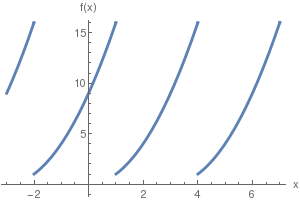Function f2(x) is defined on the interval of length 3, so ℓ = 1.5. On symmetrical interval $$(-1.5,1.5)$$ the given function can be written as
$f_2 (x) = \begin{cases} (x+3)^2 , & \ \mbox{for } -1.5 < x < 1 , \\ x^2 , & \ \mbox{for } 1 < x < 1.5 . \end{cases}$
To find its Fourier coefficients, we calculate the following integrals.
a0 = 2*Integrate[(x + 3)^2, {x, -3/2, 1/2}]/3 + 2*Integrate[x^2, {x, 1, 3/2}]/3
335/36
an = Simplify[ 2*Integrate[(x + 3)^2 * Cos[2*n*Pi*x/3], {x, -3/2, 1/2}]/3 + 2*Integrate[x^2 *Cos[n*Pi*x*2/3], {x, 1, 3/2}]/3]
(1/(4 n^3 $Pi]^3))(42 n \[Pi] Cos[(n \[Pi])/3] - 12 n \[Pi] Cos[(2 n \[Pi])/3] - 18 Sin[(n \[Pi])/3] + 49 n^2 \[Pi]^2 Sin[(n \[Pi])/3] + 18 Sin[(2 n \[Pi])/3] - 4 n^2 \[Pi]^2 Sin[(2 n \[Pi])/3] - 36 Sin[n \[Pi]] + 18 n^2 \[Pi]^2 Sin[n \[Pi]]) bn = Simplify[ 2*Integrate[(x + 3)^2 * Sin[2*n*Pi*x/3], {x, -3/2, 1/2}]/3 + 2*Integrate[x^2 *Sin[n*Pi*x*2/3], {x, 1, 3/2}]/3] (1/(4 n^3 \[Pi]^3))((18 - 49 n^2 \[Pi]^2) Cos[(n \[Pi])/3] + 2 ((-9 + 2 n^2 \[Pi]^2) Cos[(2 n \[Pi])/3] + 3 n \[Pi] (7 Sin[(n \[Pi])/3] - 2 Sin[(2 n \[Pi])/3] + 6 Sin[n \[Pi]]))) Therefore, the required Fourier series for the function f2(x) becomes \[ f_2 (x) = \frac{335}{72} + \frac{1}{4 \pi^3} \sum_{n\ge 1} \frac{1}{n^3} \left\{ 42n\pi \,\cos \frac{n\pi}{3} -12n\pi \,\cos \frac{2n\pi}{3} + \left( 49 n^2 \pi^2 -18 \right) \sin \frac{n\pi}{3} + \left( 18 - 4n^2 \pi^2 \right) \sin \frac{2n\pi}{3} \right\} \cos \frac{2n\pi x}{3} + \frac{1}{4 \pi^3} \sum_{n\ge 1} \frac{1}{n^3} \left\{ \left( 18 -49 n^2 \pi^2 \right) \cos \frac{n\pi}{3} + 2 \left( 2n^2 \pi^2 -9 \right) \cos \frac{2n\pi}{3} + 42 n\pi\,\sin \frac{n\pi}{3} - 4\,\sin \frac{2n\pi}{3} \right\} \sin \frac{2n\pi x}{3} .$
Now we build its Fourier approximation
fourier[m_] = a0/2 + Sum[an*Cos[2*n*Pi*x/3] + bn*Sin[2*n*Pi*x/3] , {n, 1, m}];
ff10[x_] = 335/72 + Sum[an*Cos[2*n*Pi*x/3] + bn*Sin[2*n*Pi*x/3], {n, 1, 10}];
ff20[x_] = 335/72 + Sum[an*Cos[2*n*Pi*x/3] + bn*Sin[2*n*Pi*x/3], {n, 1, 20}];
and plot the corresponding sums with N = 10 and 20 terms:
Plot[{ff10[x], ff20[x]}, {x, -2, 5}, PlotStyle -> {{Thick, Blue}, {Thick, Orange}}]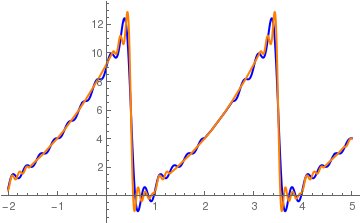•

• $$\displaystyle f_3 (x) = \begin{cases} x , & \ \mbox{for } 0 < x < 2 , \\ 1, & \ \mbox{when } -2 < x < 0 . \end{cases}$$   This function maps the finite interval (-2,2) into $$\mathbb{R} .$$

We calculate the corresponding Fourier coefficients using Mathematica:

a0 = Integrate[1, {x, -2, 0}]/2 + Integrate[x, {x, 0, 2}]/2
2
an = Simplify[ Integrate[Cos[n*Pi*x/2], {x, -2, 0}]/2 + Integrate[x *Cos[n*Pi*x/2], {x, 0, 2}]/2]
(-2 + 2 Cos[n $Pi]] + 3 n \[Pi] Sin[n \[Pi]])/(n^2 \[Pi]^2) bn = Simplify[ Integrate[Sin[n*Pi*x/2], {x, -2, 0}]/2 + Integrate[x *Sin[n*Pi*x/2], {x, 0, 2}]/2] -((n \[Pi] + n \[Pi] Cos[n \[Pi]] - 2 Sin[n \[Pi]])/(n^2 \[Pi]^2)) Therefore, the Fourier series becomes \[ f_3 (x) = 1 - \frac{4}{\pi^2} \sum_{k\ge 0} \frac{1}{(2k+1)^2} \,\cos \frac{(2k+1) \pi x}{2} - \frac{1}{\pi} \sum_{k\ge 1} \frac{1}{k}\,\sin (k\pi x) .$
Finally we build partial Fourier sums with 10 and 50 terms
ff10[x_] = 1 + 4/Pi^2 * Sum[1/(2*k+1)^2 * Cos[(2*k+1)*Pi*x/2], {k,0,10}] - Sum[1/k*Sin[k*Pi*x], {k,1,10}]/Pi; ff50[x_] = 1 + 4/Pi^2 * Sum[1/(2*k+1)^2 * Cos[(2*k+1)*Pi*x/2], {k,0,50}] - Sum[1/k*Sin[k*Pi*x], {k,1,50}]/Pi;
and plot these approximations
Plot[{ff10[x], ff50[x]}, {x, -2, 5}, PlotStyle -> {{Thick, Blue}, {Thick, Orange}}]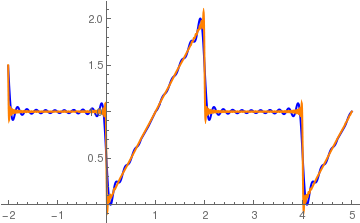Since f3(0) = ½, we get a curious sum:
$\sum_{k\ge 0} \frac{1}{(2k+1)^2} = \frac{\pi^2}{8} \approx 1.2337.$
Mathematica also knows this sum:
Sum[1/(2*k + 1)^2, {k, 0, Infinity}]
$Pi]^2/8 • • $$f_4 (x) = 1-|x|\,: \, (-2,2] \mapsto \, \mathbb{R} .$$ We first the corresponding Fourier coefficients a0 = Integrate[(1-Abs[x]), {x, -2, 2}]/2 0 an = Simplify[ Integrate[(1-Abs[x])*Cos[n*Pi*x/2], {x, -2, 2}]/2] (4 - 4 Cos[n \[Pi]] - 2 n \[Pi] Sin[n \[Pi]])/(n^2 \[Pi]^2) bn = Simplify[ Integrate[(1-Abs[x])*Sin[n*Pi*x/2], {x, -2, 2}]/2] 0 Therefore, the given function f4 has the following Fourier cosine series \[ f_4 (x) = \frac{8}{\pi^2} \sum_{k\ge 0} \frac{1}{(2k+1)^2} \,\cos \frac{(2k+1) \pi x}{2} .$
Using Mathematica, we construct partial Fourier cosine sums and the periodic extension of the given function.
ff10[x_] = 8/Pi^2 * Sum[1/(2*k+1)^2 * Cos[(2*k+1)*Pi*x/2], {k,0,10}];
ff50[x_] = 8/Pi^2 * Sum[1/(2*k+1)^2 * Cos[(2*k+1)*Pi*x/2], {k,0,50}];
per[x_] = Which[-2 Then we plot the approximation with N = 10 terms along with the given function:
Plot[{ff10[x], per[x]}, {x, -2, 5}, PlotStyle -> {{Thick, Blue}, {Thick, Orange}}]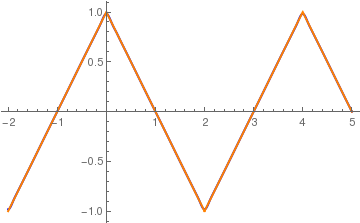•

• $$f_5 (x) = \lfloor x \rfloor \,: \, (-2,2] \mapsto \, \mathbb{R} ,$$    where ⌊x⌋ is the floor function of x. Using its Fourier coefficients
a0 = Integrate[Floor[x], {x, -2, 2}]/2
-1
an = Simplify[ Integrate[Floor[x]*Cos[n*Pi*x/2], {x, -2, 2}]/2]
-(Sin[n $Pi]]/(n \[Pi])) bn = Simplify[ Integrate[Floor[x]*Sin[n*Pi*x/2], {x, -2, 2}]/2] (4 (2 + 3 Cos[(n \[Pi])/2]) Sin[(n \[Pi])/4]^2)/(n \[Pi]) Hence, we get its Fourier series \[ f_5 (x) = - \frac{1}{2} + \frac{4}{\pi} \sum_{n\ge 1} \frac{1}{n} \left[ 2 + 3\,\cos \frac{n\pi}{2} \right] \sin^2 \frac{n\pi}{4} \,\sin \frac{n\pi x}{2} .$
Since the given function is a piecewise step functions, its Fourier series converges slowly showing Gibbs phenomenon at points of discontinuity (integer values).
ff10[x_] = -1/2 +4/Pi * Sum[1/n * (2 + 3*Cos[n*Pi/2])*(Sin[n*Pi/4])^2 * Sin[n*Pi*x/2], {n,1,10}];
ff50[x_] = -1/2 +4/Pi * Sum[1/n * (2 + 3*Cos[n*Pi/2])*(Sin[n*Pi/4])^2 * Sin[n*Pi*x/2], {n,1,50}];
per[x_] = Which[-2< x < 2,Floor[x], 2 < x < 6,Floor[x-4]]
Then we plot the approximation with N = 10 (blue) and 50 (orange) terms along with the given function (in black)):
Plot[{ff10[x], ff50[x], per[x]}, {x, -2, 5}, PlotStyle -> {{Thick, Blue}, {Thick, Orange}, {Thick, Black}}]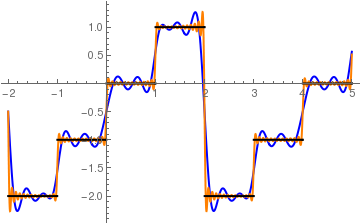We describe periodic extension and its Fourier approximation in the following example.

Example: Consider the piecewise continuous function, defined on the interval $$[-2,2] .$$

$\left\{ \begin{array}{ll} 1 , & \ x<0 \\ x^2 , & \ x>0 \end{array} \right. \qquad\mbox{on the interval } (-2,2).$
To find its Fourier series, we calculate the corresponding Fourier coefficients:
Clear[x,n,ak1,ak2,ak,bk1,bk2,bk,Sn]
ak1 = Integrate[1/2*Cos[n*Pi*x/2], {x,-2,0}];
ak2 = Integrate[1/2*x^2 * Cos[n*Pi*x/2], {x,0,2}];
bk1 = Integrate[1/2*Sin[n*Pi*x/2], {x,-2,0}];
bk2 = Integrate[1/2*x^2 * Sin[n*Pi*x/2], {x,0,2}];
ak = FullSimplify[ak1+ak2, Element[n, Integers]]
bk = FullSimplify[bk1+bk2, Element[n, Integers]]
a0 = FullSimplify[1/2* Integrate[1, {x,-2,0}] + 1/2 * Integrate[x^2 , {x,0,2}]]
Out= (8 (-1 + (-1)^n) - (1 + 3 (-1)^n) n^2 $Pi]^2)/(n^3 \[Pi]^3) Out= (8 (-1)^n)/(n^2 \[Pi]^2) Out= 7/3 Now we define its periodic expansion. This could be achieved in many ways, and we give some approaches to find a periodic expansion. f[x_] := Which[x > 2, f[x - 2*2], x < -2, f[x + 2*2], -2 < x < 0, 1 + x - x, 0 < x < 2, x^2] Plot[f[x], {x, -5, 5}, PlotStyle -> {Thick, Black}]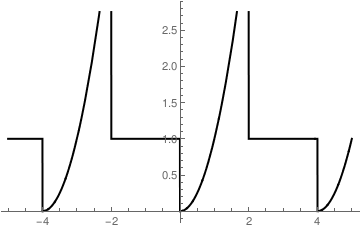Other options to define periodic function: f[x_] = Piecewise[{{1, x < 0}, {x^2, x > 0}}] Out= \[ \left\{ \begin{array}{ll} 1 & \ x<0 \\ x^2 & \ x>0 \\ 0 & \ \mbox{True} \end{array} \right.$

We define a subroutine that provide periodic extension of a function:

myperiodic[func_, {val_Symbol, min_?NumberQ, max_?NumberQ}] := func /. (val :> Mod[val - min, max - min] + min)
Then we plot it:
Plot[myperiodic[f[x], {x, -2, 2}] // Evaluate , {x, -5, 5}, PlotStyle -> {Thick, Black}]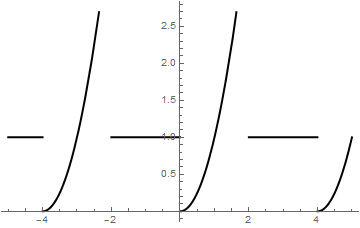We demonstrate other options to determine a periodic extension on our old piecewise continuous function.
Clear[f];
f[x_] = Piecewise[{{1, x < 0}, {x^2, x > 0}}]
f[t_ /; t >= 2] := f[t - 4]; f[t_ /; t < -2] := f[t + 4]; Plot[f[t], {t, -10, 10}] , PlotStyle -> {Thick}]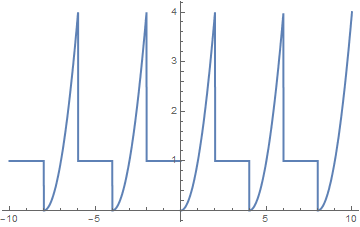f[x_] = Piecewise[{{1, x < 0}, {x^2, x > 0}}]
g[x_] = f@Mod[x, 4 , -2] (* somehow slow *)
Plot[{f[t], g[t]}, {t, -6, 6}, PlotStyle -> Thick]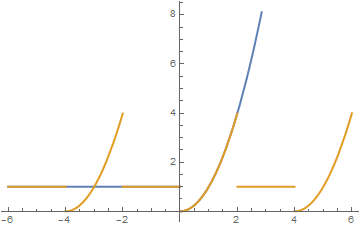A periodic extension can be defined for arbitrary period as the following example shows.
T = 2.5
g[x_ /;0<= x <= T] := x^2 +1;
f[x_] := g[Mod[x, T]]
Plot[f[t], {t, -6, 6}, PlotRange -> {{-5,5}, {-0.1, 8}}, PlotStyle -> Thick]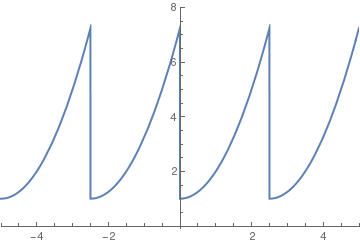Example: Consider the following function that we met previously

$f(x) = \left\{ \begin{array}{ll} \cos x, & \ \mbox{within interval [-\pi /2 , \pi /2 ]}, \\ 0 , & \ \mbox{outside the interval within } [-\pi , \pi ]. \end{array} \right.$

We can also define the periodic expansion of any function using Mod command. If we need to define a function with period $$2*\pi ,$$ we type:

periodicExtension[func_] := func[Abs[Mod[t, 2*Pi, -1*Pi]]]
per = periodicExtension[f]
Out = { Cos[Abs[Mod[t,2*Pi , -Pi]]] -Pi/2 < Abs[Mod[t, 2*Pi , -Pi]] < Pi/2
0 True
Plot[per, {t, -10, 10}, PlotStyle -> Thick]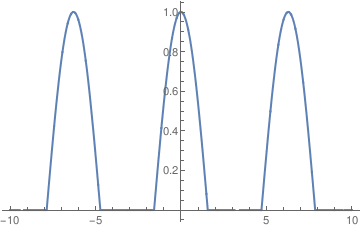FourierTrigSeries[f[t], t, 5]
Out = 1/\[Pi] + Cos[t]/2 + (2 Cos[2 t])/(3 \[Pi]) - (2 Cos[4 t])/(15 \[Pi])
Plot[%, {t, -3*Pi, 3*Pi}, PlotStyle -> Thick, AspectRatio -> 1/5]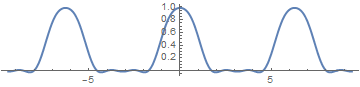1. Gregory A. Kriegsmann, A mathematical theory of full-wave rectification, IMA Journal of Applied Mathematics, Volume 36, Issue 1, 1 January 1986, Pages 29--41, https://doi.org/10.1093/imamat/36.1.29
2. C. Marouchos ; M.K. Darwish ; M. El-Habrouk, New mathematical model for analysing three-phase controlled rectifier using switching functions, IET Power Electronics, Volume: 3, Issue: 1, January 2010, 95--110. doi: 10.1049/iet-pel.2008.0328
3. Le Hong Lan and Nguyen Thi Hien, Mathematical model written by the canonical system for some electric rectifier circuits using semiconductor diodes, article
4. Branco Curgus, Fourier periodic extensions of piecewise continuous functions, http://faculty.wwu.edu/curgus/Courses/Math_pages/Math_430/Fourier_periodic_extension.html
5. Mohammed A. Abbas, Falah H.Hanoon, and Lafy F.Al-Badry, Possibility designing half-wave and full-wave molecular rectifiers by using single benzene molecule, Physics Letters A, Volume 382, Issue 8, 27 February 2018, Pages 608--612. https://doi.org/10.1016/j.physleta.2017.12.017